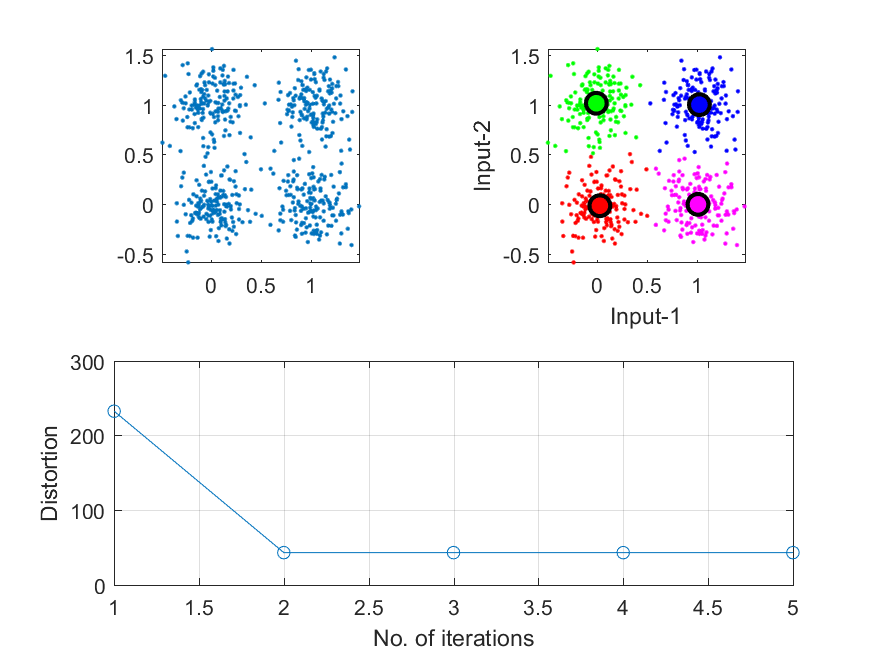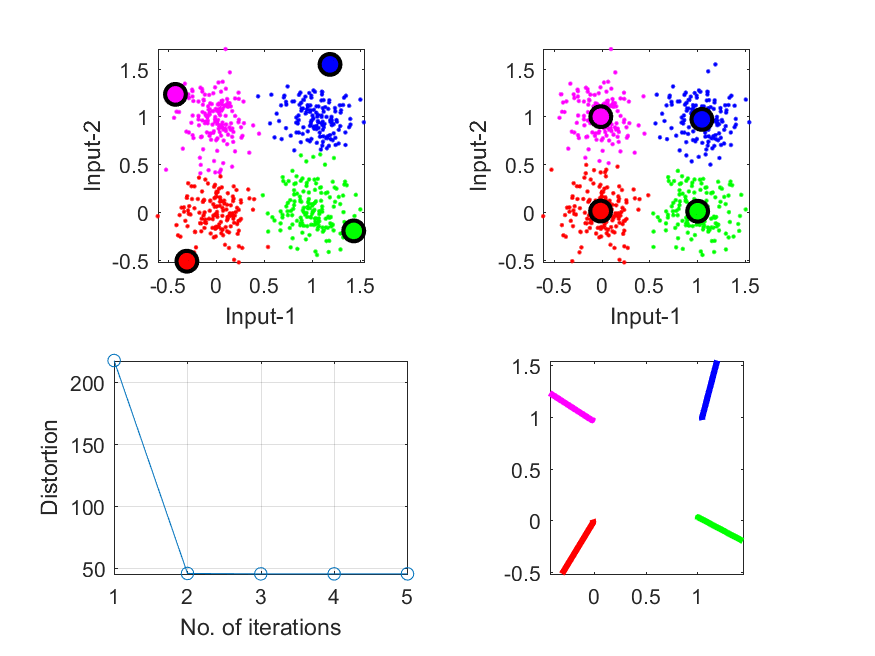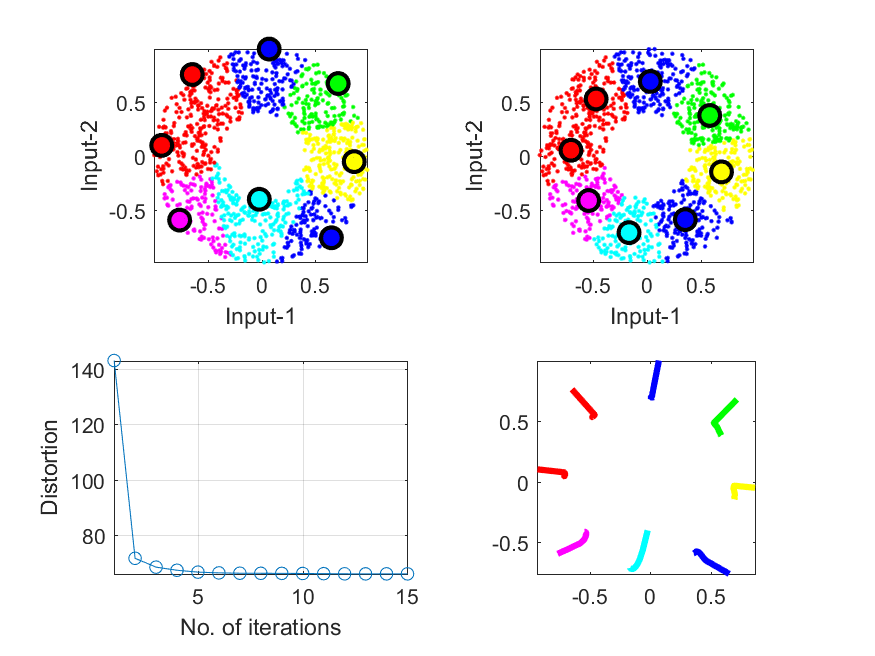## 3-3 K-means Clustering

[chinese][all]

K-means clustering (k-means for short), also known as Forgy's algorithm, is one of the most well-known methods for data clustering. The goal of k-means is to find k points of a dataset that can best represent the dataset in a certain mathematical sense (to be detailed later). These k points are also known as cluster centers, prototypes, centroids, or codewords, and so on. After obtaining these cluster centers, we can use them for numerous tasks, including:

• Data compression: We can use these cluster centers to represent the original dataset. Since the number of centers is much less than the size of the original dataset, the goal of data compression can be achieved.
• Data classification: We can use these cluster centers for data classification such that the computation load is lessened and the influence from noisy data is reduced.

K-means is also a method of partitional clustering in which we need to specify the number of clusters before starting the clustering process. Suppose that the number of clusters is m, then we can define an objective function as the sum of square distances between a data point and its nearest cluster centers. We can follow a procedure to minimize the objective function iteratively by finding a new set of cluster centers that can lower the value of the objective function at each iteration.

More specifically, suppose that we have a data set to be divided into m clusters. The data points in cluster p are represented by a set Gp with a cluster center cp. Then the square error of cluster p can be defined as $$e_j=\sum_{x_i \in G_j} \| x_i-c_j\|^2.$$

We can then define the objective function as the square error of all the m clusters:

E = Sp=1m ep

The above objective function is also referred to as the distortion when using these cluster centers to represent the whole dataset. It is obvious that the objective function depends on the cluster centers. Therefore we need to find a systematic method for identifying these clusters and their corresponding centers for minimizing E. In other words, the problem of clustering can be formulated as a problem of optimization, which requires more rigorous notation, as explained next.

Suppose that the dataset X is composed of n vectors, X = {x1, ..., xn}, where each vector is of length d. The goal of k-means is to find a way of dividing the original dataset into k clusters with cluster centers C = {c1, ..., ck} such that the following objective function is minimized:

J(X; C, A) = Sxi in Gp|xi-cp|2,
where xi belongs to Gp and cp is the centers for Gp. In the above objective function, A is an mxn membership matrix (or partition matrix) with values of 0 or 1. A(i, j) = 1 if and only if data point j belongs to cluster i. It should be noted that there should be only a single 1 in each column of A since each data point only belongs to a cluster. Moreover, it should be clear that A appears in the right-hand side of the the above equation in an implicit manner.

It would be rather difficult to optimize the above objective function directly since it involves the optimizaiton of d*m (for matrix C) plus m*n (for matrix A) tunalbe parameters with certain constraints on matrix A. However, we can observe two facts:

1. When C (cluster centers) is fixed, we can easily identify the corresponding optimizing A such that the objective function J(X; C, A) is minimized. The optimizing A is equivalent to the clustering strategy that each data point belongs to the cluster whose centers is the nearest center to this data point.
2. When A (membership matrix) is fixed, we can simply find the optimizing centers such that the objective function J(X; C, A) is minimized. Since our objective function is the square error, the minimizing center for each cluster is the mean vector of all the data points in the cluster.
According to the above observations, we can describe k-means clustering algorithm as follows:
1. Randomly select k data points as the initial centers for k clusters. These centers form the columns of C.
2. Generate the best membership matrix A based on the given C. In other words, assign a data point x to the cluster whose center is the nearest center to x.
3. Compute the objective function J(X; C, A). Stop the iteration if the objective function does not improve much from the previous iteration.
4. Generate the best C based on the given A.
5. Repeat step 2 ~ 4 until a maximum number of iterations is reached.

In the above algorithm, we set the initial centers C first and obtain A for carrying out the subsequent iterations. It is also possible to set the initial clusters A first and obtain C for carrying out subsequent iterations. The result will be similar.

At each iteration, we can prove that the value of the objective function J(X; C, A) is monotonically non-increasing based on the previous mentioned two observations. Moreover, if the centers stay the same in two consecutive iteration, then the objective funciton reaches a plateau and never changes afterwards. The following example demonstrates the use of k-means on a two-dimensional dataset:

Example 1: kMeans01.m% ====== Get the data set DS = dcData(5); subplot(2,2,1); plot(DS.input(1,:), DS.input(2,:), '.'); axis tight, axis image % ====== Run kmeans centerNum=4; [center, U, distortion, allCenters] = kMeansClustering(DS.input, centerNum); % ====== Plot the result subplot(2,2,2); vqDataPlot(DS.input, center); subplot(2,1,2); plot(distortion, 'o-'); xlabel('No. of iterations'); ylabel('Distortion'); grid onThe upper left plot in the above example shows the scatter plot of the dataset. The upper right plot shows the clustering results. The lower plot demonstrates how the objective function (distortion) decreases with each iteration. Since the initial centers are selected randomly, you are likely to get slightly different results for each run of the example.

The next example uses the same dataset for k-means and plots the trajectories of centers during the process of clustering:

Example 2: kMeans02.m% ====== Get the data set DS = dcData(5); centerNum=4; % ====== Get initial cluster centers initCenter=vqCenterInit(DS.input, centerNum); subplot(2,2,1); vqDataPlot(DS.input, initCenter); % ====== Run k-means to get the final cluster centers [center, U, distortion, allCenters] = kMeansClustering(DS.input, initCenter); subplot(2,2,2); vqDataPlot(DS.input, center); % ====== Plot the distortion subplot(2,2,3); plot(1:length(distortion), distortion, 'o-'); xlabel('No. of iterations'); ylabel('Distortion'); grid on; axis tight % ====== Plot the moving paths of the centers subplot(2,2,4); for i=1:centerNum xData=allCenters(1,i,:); xData=xData(:); yData=allCenters(2,i,:); yData=yData(:); line(xData, yData, 'color', getColor(i), 'lineWidth', 3); end box on; axis imageIn the above example:
• The upper-left plot is the inital centers and the corresponding clusters.
• The upper-right plot is the final centers and the corresponding clusters.
• The lower-left plot is the distortion with respect to the number of iterations.
• The lower-right plot is the trajectories of the centers during the clustering process.

In the above example, we can clearly identify 4 clusters by visual inspection. If we set the number of clusters to be 4 for running k-means, the result is often satisfactory. However, if there is no way for visual inspection (say, when the data dimensions are more than 3), then we need to use methods in cluster validation (see the exercises) for identify the optimum number of clusters.

The following example shows the use of k-means on another 2D dataset of a donut shape:

Example 3: kMeans03.m% ====== Get the data set DS = dcData(2); centerNum=8; % ====== Get initial cluster centers initCenter=vqCenterInit(DS.input, centerNum); clf; subplot(2,2,1); vqDataPlot(DS.input, initCenter); % ====== Run k-means to get the final cluster centers [center, U, distortion, allCenters] = kMeansClustering(DS.input, initCenter); subplot(2,2,2); vqDataPlot(DS.input, center); % ====== Plot the distortion subplot(2,2,3); plot(1:length(distortion), distortion, 'o-'); xlabel('No. of iterations'); ylabel('Distortion'); grid on; axis tight % ====== Plot the moving paths of the centers subplot(2,2,4); for i=1:centerNum xData=allCenters(1,i,:); xData=xData(:); yData=allCenters(2,i,:); yData=yData(:); line(xData, yData, 'color', getColor(i), 'lineWidth', 3); end box on; axis imageThere are some other facts of k-means that we should be aware of:

• The iteration in k-means can only guarantee the non-increasingness of the objective function J(X; C, A). However, it cannot guarantee the finding of the global minimum of the objective function. In fact, there is no efficient methods that can guarantee the finding of the global minimum of the objective function. Hence it is adviceable to run k-means multiple times starting from different initial centers and then keep the best result.
• A better set of initial centers will have positive influence on the final clustering results. Therefore it is an important issue to be able to rapidly select some good initial centers heuristically. Some commonly used methods for initial center selection include:
1. Randomly select m data points from the dataset.
2. Select the farthest m data points from the mean of the dataset.
3. Select the nearest m data points from the mean of the dataset.
4. Select m data points that have the largest sum of pairwise square distance.
Intuitively, the last method can usually selet a better set of initial centers at the cost of more computation. But again, there is no guarantee as which method can always generate the better result.
• During the iteration of k-means, it could happen that a cluster is formed with no elements at all. This is more likely to happen for dataset of higher dimensions. Once we encounter such a situation, the most straightforward method is to remove the empty cluster and then split another cluster into two such that the total number of clusters is the same. As the selection criteria for cluster to be split, we have at least two:
1. Select the cluster that contributes most to the objective function.
2. Select the cluster with the maximal number of data points.

The k-means method we have discussed so far is also referred to as the batch k-means algorithm. Another similar method for on-line clustering is called the sequential/online k-means algorithm. The sequential version updates the centers whenever a data point x is available, as follows:

1. Randomly select m data points as the initial centers for m clusters.
2. Find the cluster center that is nearest to the incoming data point x. Add the data point x to the cluster and update the cluster center as the mean vector of all the data points in this cluster.
3. Check if the nearest center of a data point is the center this data point belongs to. Repeat to check all the data points. If the answer is yes for all the data points, stop the iteration. Otherwise go back to step 2.

In general, we have the following two situations for using the sequential k-mean algorithm:

• Suitable for time varying system where the characteristics of the dataset is varying with time.
• Suitable for low-end platform with less computing power.
In practice, we seldom use the sequential k-mean algorithm unless for the two reasons stated above.
Data Clustering and Pattern Recognition (資料分群與樣式辨認)